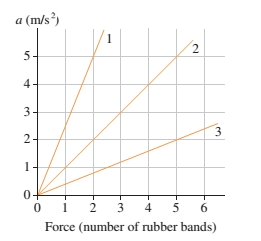# Problem: Figure 1 shows an acceleration-versus-force graph for three objects pulled by rubber bands. The mass of object 2 is 0.40kg.Part A) What is the mass of object 1?Part B) What is the mass of object 3?

###### FREE Expert Solution

Newton's second law:

$\overline{){\mathbf{F}}{\mathbf{=}}{\mathbf{m}}{\mathbf{a}}}$

Part A

F1 = m1a1

F2 = m2a2

F1/F2 = (m1a1)/(m2a2)

86% (369 ratings)###### Problem Details

Figure 1 shows an acceleration-versus-force graph for three objects pulled by rubber bands. The mass of object 2 is 0.40kg.Part A) What is the mass of object 1?

Part B) What is the mass of object 3?